Version: 2018.4
Language : English
Accessing shader properties in Cg/HLSL
Built-in shader include files

# Providing vertex data to vertex programs

For Cg/HLSL vertex programs, the vertex data is passed as inputs to the vertex shader function. Each input needs to have semantic speficied for it: for example, `POSITION` input is the vertex position, and `NORMAL` is the vertex normal.

Often, vertex data inputs are declared in a structure, instead of listing them one by one. Several commonly used vertex structures are defined in UnityCG.cginc include file, and in most cases it’s enough just to use those. The structures are:

• `appdata_base`: position, normal and one texture coordinate.
• `appdata_tan`: position, tangent, normal and one texture coordinate.
• `appdata_full`: position, tangent, normal, four texture coordinates and color.

Example: This colors the mesh based on its normals, and uses `appdata_base` as vertex program input:

``````Shader "VertexInputSimple" {
__SubShader__Each shader in Unity consists of a list of subshaders. When Unity has to display a mesh, it will find the shader to use, and pick the first subshader that runs on the user's graphics card. [More info](SL-SubShader.html)<span class="tooltipGlossaryLink">See in [Glossary](Glossary.html#subshader)</span> {
Pass {
CGPROGRAM
#pragma vertex vert
#pragma fragment frag
#include "UnityCG.cginc"

struct v2f {
float4 pos : SV_POSITION;
fixed4 color : COLOR;
};

v2f vert (appdata_base v)
{
v2f o;
o.pos = UnityObjectToClipPos(v.vertex);
o.color.xyz = v.normal * 0.5 + 0.5;
o.color.w = 1.0;
return o;
}

fixed4 frag (v2f i) : SV_Target { return i.color; }
ENDCG
}
}
}
``````

To access different vertex data, you need to declare the vertex structure yourself, or add input parameters to the vertex shader. Vertex data is identified by Cg/HLSL semantics, and must be from the following list:

• `POSITION` is the vertex position, typically a `float3` or `float4`.
• `NORMAL` is the vertex normal, typically a `float3`.
• `TEXCOORD0` is the first UV coordinate, typically `float2`, `float3` or `float4`.
• `TEXCOORD1`, `TEXCOORD2` and `TEXCOORD3` are the 2nd, 3rd and 4th UV coordinates, respectively.
• `TANGENT` is the tangent vector (used for normal mapping), typically a `float4`.
• `COLOR` is the per-vertex color, typically a `float4`.

When the mesh data contains fewer components than are needed by the vertex shader input, the rest are filled with zeroes, except for the `.w` component which defaults to 1. For example, mesh texture coordinates are often 2D vectors with just x and y components. If a vertex shader declares a `float4` input with `TEXCOORD0` semantic, the value received by the will contain (x,y,0,1).

## Examples

### Visualizing UVs

The following shader example uses the vertex position and the first texture coordinate as the vertex shader inputs (defined in the structure appdata). This shader is very useful for debugging the UV coordinates of the mesh.

``````Shader "Debug/UV 1" {
SubShader {
Pass {
CGPROGRAM
#pragma vertex vert
#pragma fragment frag
#include "UnityCG.cginc"

// vertex input: position, UV
struct appdata {
float4 vertex : POSITION;
float4 texcoord : TEXCOORD0;
};

struct v2f {
float4 pos : SV_POSITION;
float4 uv : TEXCOORD0;
};

v2f vert (appdata v) {
v2f o;
o.pos = UnityObjectToClipPos(v.vertex );
o.uv = float4( v.texcoord.xy, 0, 0 );
return o;
}

half4 frag( v2f i ) : SV_Target {
half4 c = frac( i.uv );
if (any(saturate(i.uv) - i.uv))
c.b = 0.5;
return c;
}
ENDCG
}
}
}
``````

Here, UV coordinates are visualized as red and green colors, while an additional blue tint has been applied to coordinates outside of the 0 to 1 range: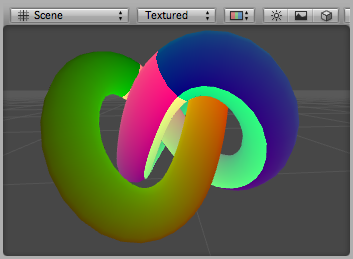Debug UV1 shader applied to a torus knot model

Similarly, this shader vizualizes the second UV set of the model:

``````Shader "Debug/UV 2" {
SubShader {
Pass {
CGPROGRAM
#pragma vertex vert
#pragma fragment frag
#include "UnityCG.cginc"

// vertex input: position, second UV
struct appdata {
float4 vertex : POSITION;
float4 texcoord1 : TEXCOORD1;
};

struct v2f {
float4 pos : SV_POSITION;
float4 uv : TEXCOORD0;
};

v2f vert (appdata v) {
v2f o;
o.pos = UnityObjectToClipPos(v.vertex );
o.uv = float4( v.texcoord1.xy, 0, 0 );
return o;
}

half4 frag( v2f i ) : SV_Target {
half4 c = frac( i.uv );
if (any(saturate(i.uv) - i.uv))
c.b = 0.5;
return c;
}
ENDCG
}
}
}
``````

### Visualizing vertex colors

The following shader uses the vertex position and the per-vertex colors as the vertex shader inputs (defined in structure appdata).

``````Shader "Debug/Vertex color" {
SubShader {
Pass {
CGPROGRAM
#pragma vertex vert
#pragma fragment frag
#include "UnityCG.cginc"

// vertex input: position, color
struct appdata {
float4 vertex : POSITION;
fixed4 color : COLOR;
};

struct v2f {
float4 pos : SV_POSITION;
fixed4 color : COLOR;
};

v2f vert (appdata v) {
v2f o;
o.pos = UnityObjectToClipPos(v.vertex );
o.color = v.color;
return o;
}

fixed4 frag (v2f i) : SV_Target { return i.color; }
ENDCG
}
}
}
``````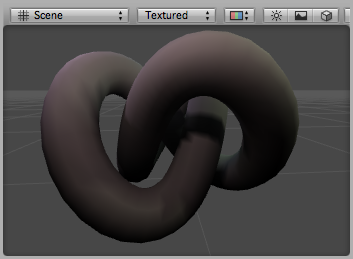Debug Colors shader applied to a torus knot model that has illumination baked into colors

### Visualizing normals

The following shader uses the vertex position and the normal as the vertex shader inputs (defined in the structure appdata). The normal’s X,Y & Z components are visualized as RGB colors. Because the normal components are in the –1 to 1 range, we scale and bias them so that the output colors are displayable in the 0 to 1 range.

``````Shader "Debug/Normals" {
SubShader {
Pass {
CGPROGRAM
#pragma vertex vert
#pragma fragment frag
#include "UnityCG.cginc"

// vertex input: position, normal
struct appdata {
float4 vertex : POSITION;
float3 normal : NORMAL;
};

struct v2f {
float4 pos : SV_POSITION;
fixed4 color : COLOR;
};

v2f vert (appdata v) {
v2f o;
o.pos = UnityObjectToClipPos(v.vertex );
o.color.xyz = v.normal * 0.5 + 0.5;
o.color.w = 1.0;
return o;
}

fixed4 frag (v2f i) : SV_Target { return i.color; }
ENDCG
}
}
}
``````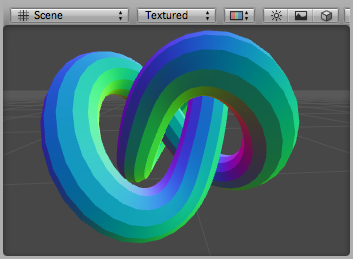Debug Normals shader applied to a torus knot model. You can see that the model has hard shading edges.

### Visualizing tangents and binormals

Tangent and binormal vectors are used for normal mapping. In Unity only the tangent vector is stored in vertices, and the binormal is derived from the normal and tangent values.

The following shader uses the vertex position and the tangent as vertex shader inputs (defined in structure appdata). Tangent’s x,y and z components are visualized as RGB colors. Because the normal components are in the –1 to 1 range, we scale and bias them so that the output colors are in a displayable 0 to 1 range.

``````Shader "Debug/Tangents" {
SubShader {
Pass {
CGPROGRAM
#pragma vertex vert
#pragma fragment frag
#include "UnityCG.cginc"

// vertex input: position, tangent
struct appdata {
float4 vertex : POSITION;
float4 tangent : TANGENT;
};

struct v2f {
float4 pos : SV_POSITION;
fixed4 color : COLOR;
};

v2f vert (appdata v) {
v2f o;
o.pos = UnityObjectToClipPos(v.vertex );
o.color = v.tangent * 0.5 + 0.5;
return o;
}

fixed4 frag (v2f i) : SV_Target { return i.color; }
ENDCG
}
}
}
``````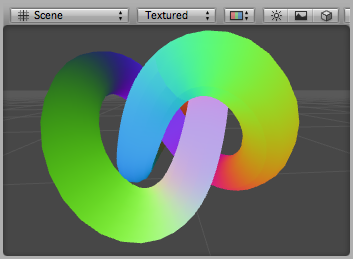Debug Tangents shader applied to a torus knot model.

The following shader visualizes bitangents. It uses the vertex position, normal and tangent values as vertex inputs. The bitangent (sometimes called binormal) is calculated from the normal and tangent values. It needs to be scaled and biased into a displayable 0 to 1 range.

``````Shader "Debug/Bitangents" {
SubShader {
Pass {
Fog { Mode Off }
CGPROGRAM
#pragma vertex vert
#pragma fragment frag
#include "UnityCG.cginc"

// vertex input: position, normal, tangent
struct appdata {
float4 vertex : POSITION;
float3 normal : NORMAL;
float4 tangent : TANGENT;
};

struct v2f {
float4 pos : SV_POSITION;
float4 color : COLOR;
};

v2f vert (appdata v) {
v2f o;
o.pos = UnityObjectToClipPos(v.vertex );
// calculate bitangent
float3 bitangent = cross( v.normal, v.tangent.xyz ) * v.tangent.w;
o.color.xyz = bitangent * 0.5 + 0.5;
o.color.w = 1.0;
return o;
}

fixed4 frag (v2f i) : SV_Target { return i.color; }
ENDCG
}
}
}
``````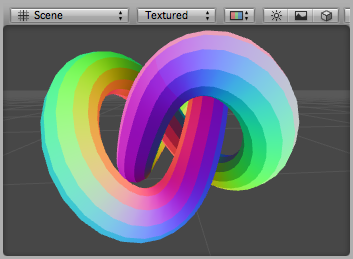Debug Bitangents shader applied to a torus knot model.

## Further Reading

Accessing shader properties in Cg/HLSL
Built-in shader include files